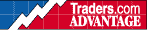HOT TOPICS LIST

INDICATORS LIST

LIST OF TOPICS

# Chaikin Oscillator

07/31/00 05:34:52 PM
by Jason K. Hutson

Here are the Excel formulas for the Chaikin indicators using daily price data for Paramount.

Security:   N/A
Position:   N/A

 In column F, the single day's measurement of money flow is calculated. The following formula is entered into cell F2 and copied down:=(((D2-C2)-(B2-D2))/(B2-C2))*E2Column E is VolumeColumn D is CloseColumn C is LowColumn B is HighThe cumulative line is calculated in column G. Start with the first day's value of the single day's money flow by entering the following formula into cell G1:=F2 Then start the running total in cell G3 by entering the following formula in cell G3 and copying down:=F3+G2The Chaikin oscillator is the difference between the three-period simple moving average and the 10-period simple moving average of the cumulative line. The three-period simple moving average is calculated in column H. The following formula is entered into cell H4 and is copied down:=AVERAGE(G2:G4) The 10-period simple moving average of the cumulative line is calculated in column I. Enter the following formula into cell I11 and copy down:=AVERAGE(G2:G11) The Chaikin oscillator is calculated in column J. The following formula is entered into cell J11 and is copied down:=H11-I11This article taken in part from:Stocks & Commodities V. 12:1 (30-37): SIDEBAR: CHAIKIN INDICATORS

Jason K. Hutson

Traders.com Staff Writer. Enjoys trendlines, support and resistance, moving averages, RSI, MACD, ADX, Bollinger bands, parabolic SAR, chart formations, and volume analysis.

 Comments or Questions? Article Usefulness 5 (most useful) 4 3 2 1 (least useful)

Date:�/ /Rank:�5Comment: